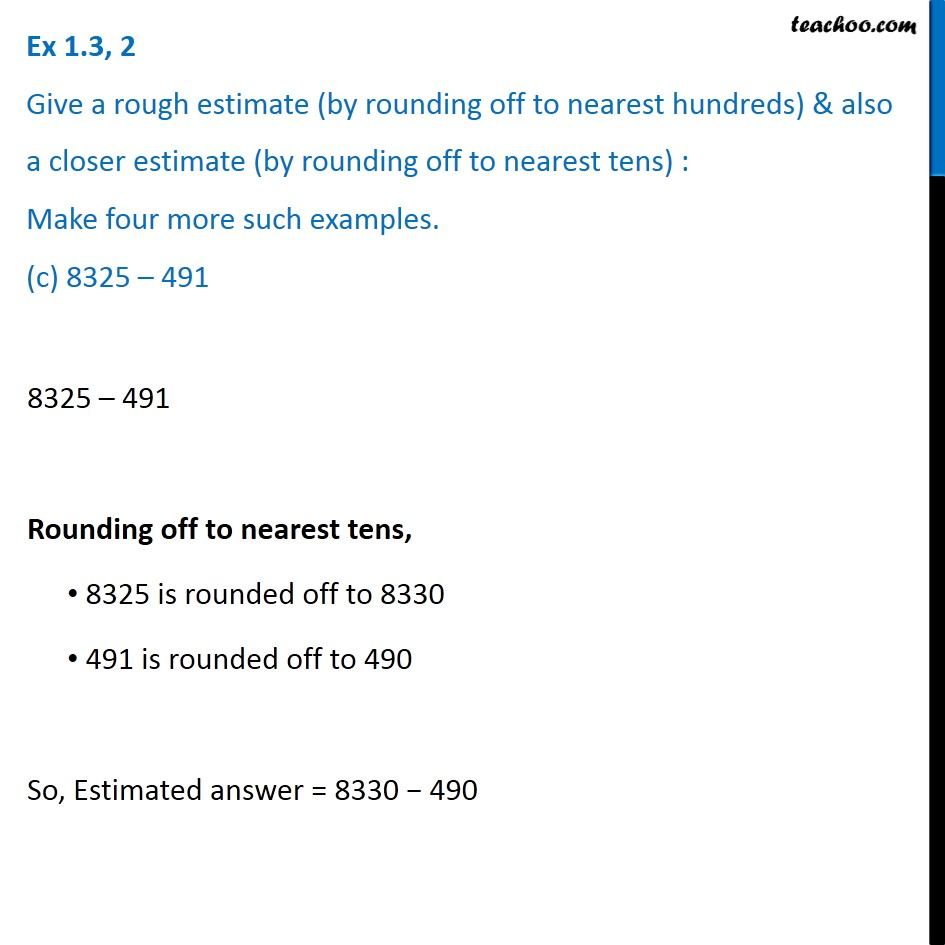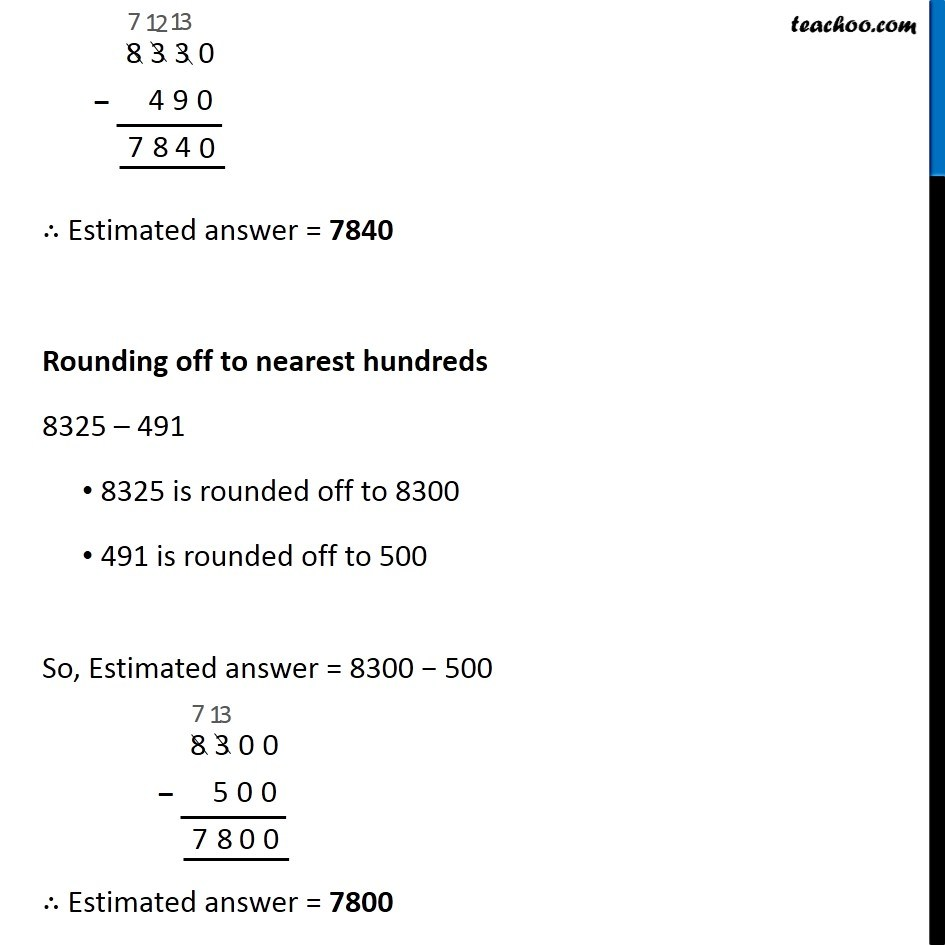1. Chapter 1 Class 6 Knowing our Numbers
2. Serial order wise
3. Ex 1.3

Transcript

Ex 1.3, 2 Give a rough estimate (by rounding off to nearest hundreds) & also a closer estimate (by rounding off to nearest tens) : Make four more such examples. (c) 8325 – 4918325 – 491 Rounding off to nearest tens, 8325 is rounded off to 8330 491 is rounded off to 490 So, Estimated answer = 8330 − 490 ∴ Estimated answer = 7,840 Rounding off to nearest hundreds 8325 is rounded off to 8300 491 is rounded off to 500 So, Estimated answer = 8300 − 500 ∴ Estimated answer = 7800